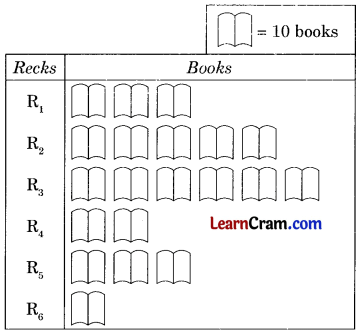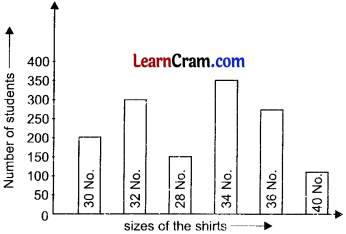# DAV Class 6 Maths Chapter 16 Brain Teasers Solutions

The DAV Class 6 Maths Book Solutions and DAV Class 6 Maths Chapter 16 Brain Teasers Solutions of Statistics offer comprehensive answers to textbook questions.

## DAV Class 6 Maths Ch 16 Brain Teasers Solutions

### DAV Class 6 Maths Chapter 16 HOTS

Question 1.
Below is given a bar graph showing the production of wheat and rice in different states of India. Read the bar graph and answer the question given below:(a) What information is given in the bar graph?
(b) Which state is the largest producer of rice?
(c) Which state has total production of rice and wheat as its maximum?
(d) What percent is the production of rice and wheat in Maharashtra over the production of rice and wheat in MP?
(e) Which states have total production of rice and wheat same?
(a) The production of rice and wheat in five states of India.
(b) West Bengal.
(c) U.P.
(d) Production of wheat and rice in Maharashtra = 4 + 2 = 6
Production of wheat and rice in MP = 6 + 6 = 12
Difference in production = 12 – 6 = 6
Percent increase = 6/12 × 100 = 50%
(e) West Bengal (10 + 2 = 12) and Madhya Pradesh (6 + 6 = 12).

Question 1.
Number of books placed in different recks in a library is given below.Observe the above table and answer the following questions.
(a) How many books are placed in R1?
(b) Which reck has the maximum books?
(c) Which reck has the minimum books?
(d) Total number of books in the library.
(a) Number of books placed in R1 = 3 × 10 = 30 books.
(b) R3 has 6 × 10 = 60 books which is maximum number of books.
(c) R6 has minimum number of books i.e. 1 × 10 = 10 books.
(d) Total number of books is 20 × 10 = 200 books.Question 2.
The following bar graph shows the number of students wearing shirts of different sizes.Observe the above bar graph and answer the following questions:
(a) HOWT many students wear 32 No. shirts?
Number of students who wear 32 No. shirts = 300

(b) How many students wear the largest No. shirts?
Number of students who wear the largest No. of shirts = 100

(c) 1 low many students wear the smallest No. of shirts?
Number of students who wear the smallest No. of shirts = 150

(d) WThat is the range of shirts No.?